# How to Calculate and Solve for Linear Flow Rate in Reservoir Fluid Flow | The Calculator Encyclopedia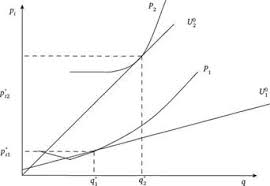The image above represents the linear flow rate.

To compute for the linear flow rate, four parameters are needed and these parameters are Initial pressure (P1), Final Pressure (P2), Thickness (h) and Permeability (k).

The formula for calculating linear flow rate:

q = 0.001127kh[P1 – P2]

Where:
q = Linear Flow Rate
P1 = Initial Pressure
P2 = Final Pressure
h = Thickness
k = Permeability

Let’s solve an example;
Find the linear flow rate when the initial pressure is 12, final pressure is 22, thickness is 18 and permeability is 44.

This implies that;
P1 = Initial Pressure = 12
P2 = Final Pressure = 22
h = Thickness = 18
k = Permeability = 44

q = 0.001127kh [P1 – P2]
q = 0.001127 x 44 x 18 [12 – 22]
q = 0.001127 x 44 x 18 [-10]
q = 0.892584 [-10]
q = -8.92584

Therefore, the linear flow rate is -8.92584 bbl/day.

Calculating Permeability when the linear flow rate, Initial Pressure, Final Pressure and Thickness is Given.

k = q / 0.001127h (p1 – p2)

Where;
k = Permeability
q = Linear Flow Rate
P1 = Initial Pressure
P2 = Final Pressure
h = Thickness

Let’s solve an example;
Find the permeability when the initial pressure is 34, final pressure is 24, thickness is 12 and linear flow rate is 50.

This implies that;
q = Linear Flow Rate = 50
P1 = Initial Pressure = 34
P2 = Final Pressure = 24
h = Thickness = 12

k = q / 0.001127h (p1 – p2)
k = 50 / 0.001127h (34 – 24)
k = 50 / 0.001127h (10)
k = 50 / 0.01127
k = 4436.5

Therefore, the permeability is 4436.5.

Calculating Thickness when the linear flow rate, Initial Pressure, Final Pressure and Permeability is Given.

h = q / 0.001127k (p1 – p2)

Where;
h = Thickness
k = Permeability
q = Linear Flow Rate
P1 = Initial Pressure
P2 = Final Pressure

Let’s solve an example;
Find the thickness when the initial pressure is 24, final pressure is 12, permeability is 20 and linear flow rate is 30.

This implies that;
k = Permeability = 20
q = Linear Flow Rate = 30
P1 = Initial Pressure = 24
P2 = Final Pressure = 12

h = q / 0.001127k (p1 – p2)
h = 30 / 0.001127 x 20 (24 – 12)
h = 30 / 0.02254 (12)
h = 30 / 0.27048
h = 110.9

Therefore, the thickness is 110.9.

Calculating Initial Pressure when the linear flow rate, Thickness , Final Pressure and Permeability is Given.

p1 = q / 0.001127kh + p2

Where;
P1 = Initial Pressure
q = Linear Flow Rate
h = Thickness
k = Permeability
P2 = Final Pressure

Let’s solve an example;
Find the initial pressure when the thickness is 16, final pressure is 20, permeability is 24 and linear flow rate is 70.

This implies that;
q = Linear Flow Rate = 70
h = Thickness = 16
k = Permeability = 24
P2 = Final Pressure = 20

p1 = q / 0.001127kh + p2
p1 = 70 / 0.001127 x 24 x 16 + 20
p1 = 70 / 20.432768
p1 = 3.425

Therefore, the initial pressure is 3.425.

Calculating Final Pressure when the linear flow rate, Thickness , Initial Pressure and Permeability is Given.

p2 = p1q / 0.001127kh

Where;
P2 = Final Pressure
P1 = Initial Pressure
q = Linear Flow Rate
h = Thickness
k = Permeability

Let’s solve an example;
Find the final pressure when the thickness is 15, initial pressure is 28, permeability is 17 and linear flow rate is 56.

This implies that;
P1 = Initial Pressure = 28
q = Linear Flow Rate = 56
h = Thickness = 15
k = Permeability = 17

p2 = p1q / 0.001127kh
p2 = 28 – 56 / 0.001127 x 15 x 17
p2 = 28 – 56 / 0.28735
p2 = 28 – 194.88
p2 = -166.88

Therefore, the final pressure is -166.88.

Nickzom Calculator – The Calculator Encyclopedia is capable of calculating the linear flow rate

To get the answer and workings of the linear flow rate using the Nickzom Calculator – The Calculator Encyclopedia. First, you need to obtain the app.

You can get this app via any of these means:

To get access to the professional version via web, you need to register and subscribe for NGN 1,500 per annum to have utter access to all functionalities.
You can also try the demo version via https://www.nickzom.org/calculator

Apple (Paid) – https://itunes.apple.com/us/app/nickzom-calculator/id1331162702?mt=8
Once, you have obtained the calculator encyclopedia app, proceed to the Calculator Map, then click on Petroleum under EngineeringNow, Click on Reservoir Fluid Flow under Petroleum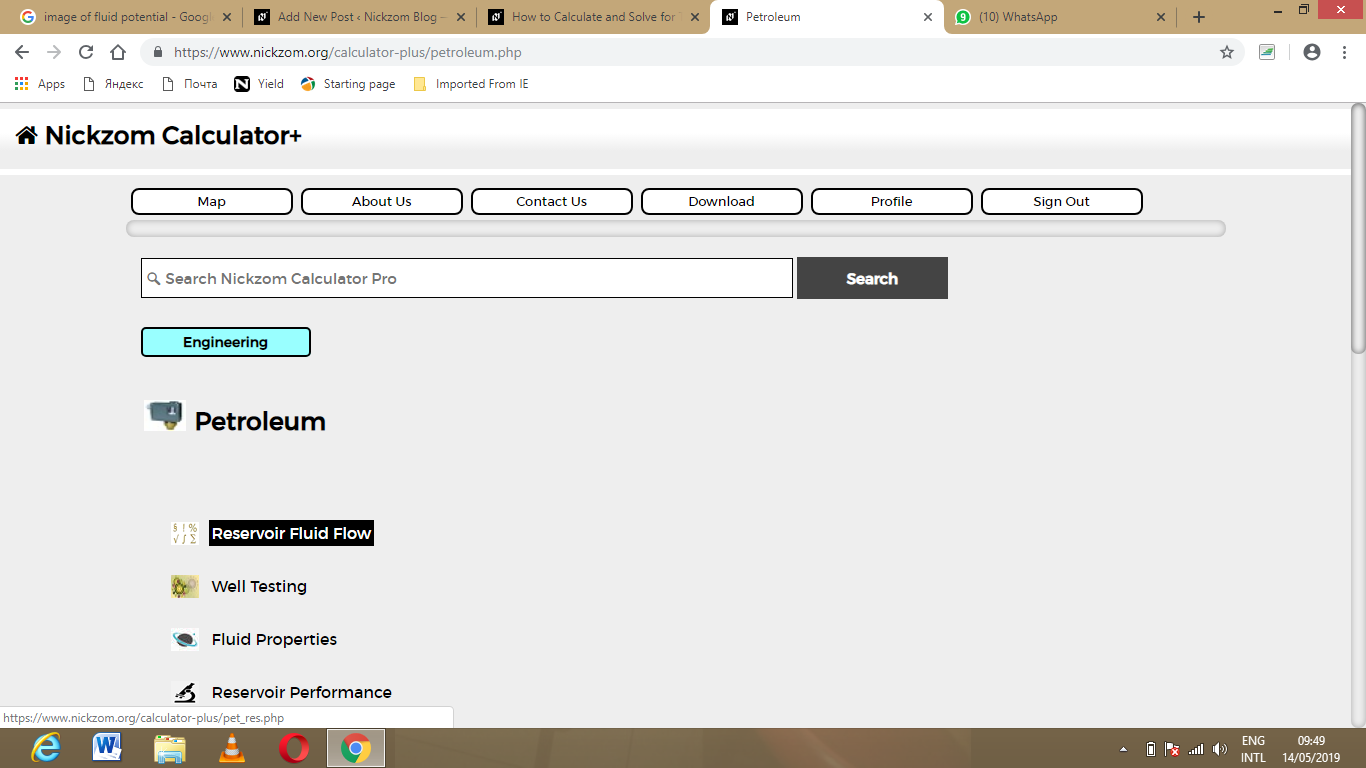Now, Click on Linear Flow Rate under Reservoir Fluid Flow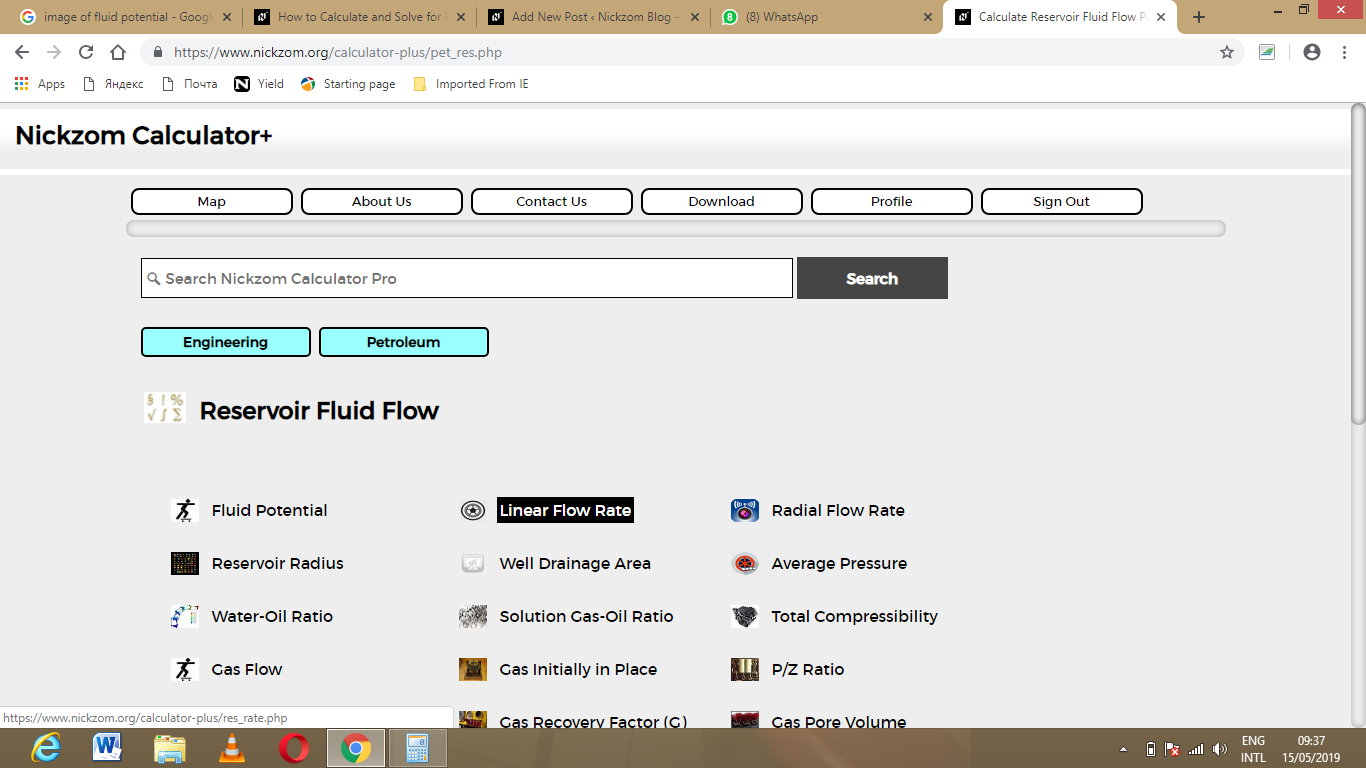The screenshot below displays the page or activity to enter your value, to get the answer for the linear flow rate according to the respective parameter which are the Initial pressure (P1), Final Pressure (P2), Thickness (h) and Permeability (k).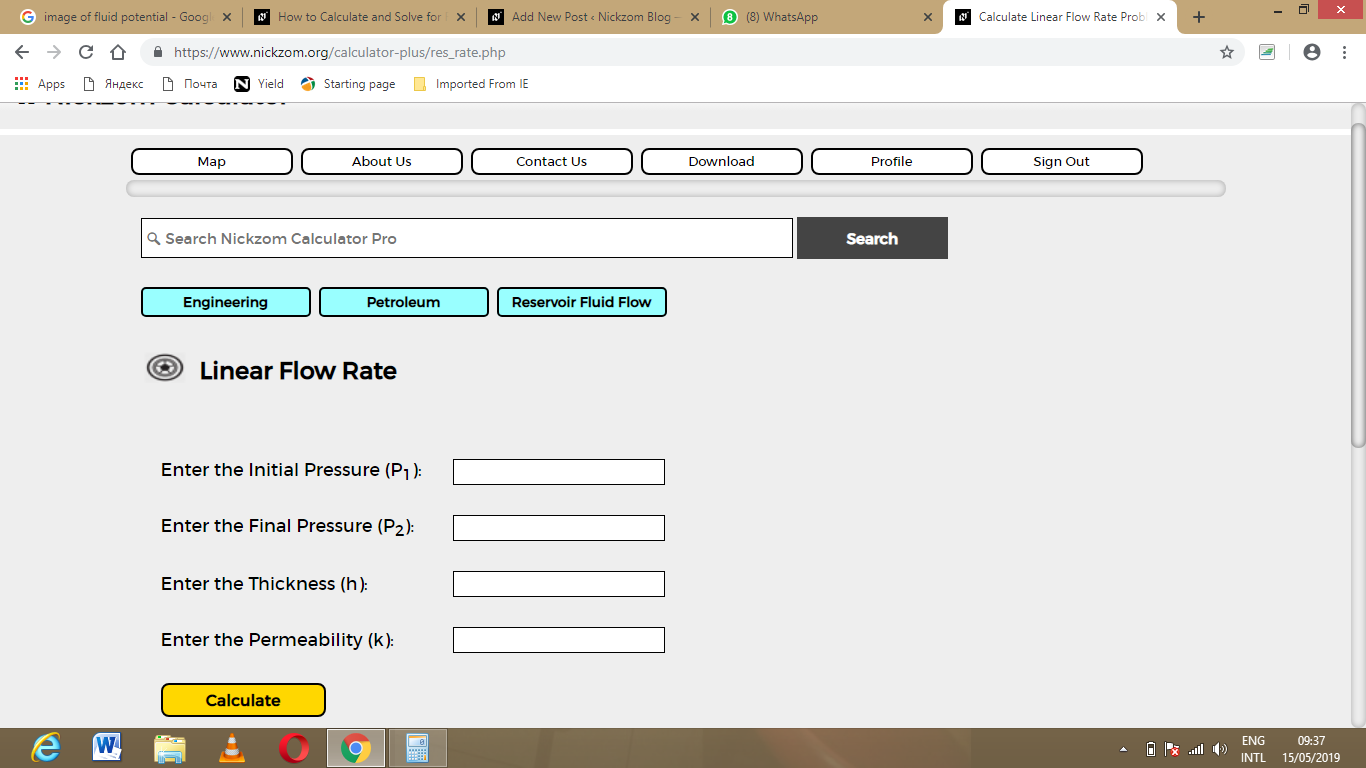Now, enter the value appropriately and accordingly for the parameter as required by the Initial pressure (P1) is 12, Final Pressure (P2) is 22, Thickness (h) is 18 and Permeability (k) is 44.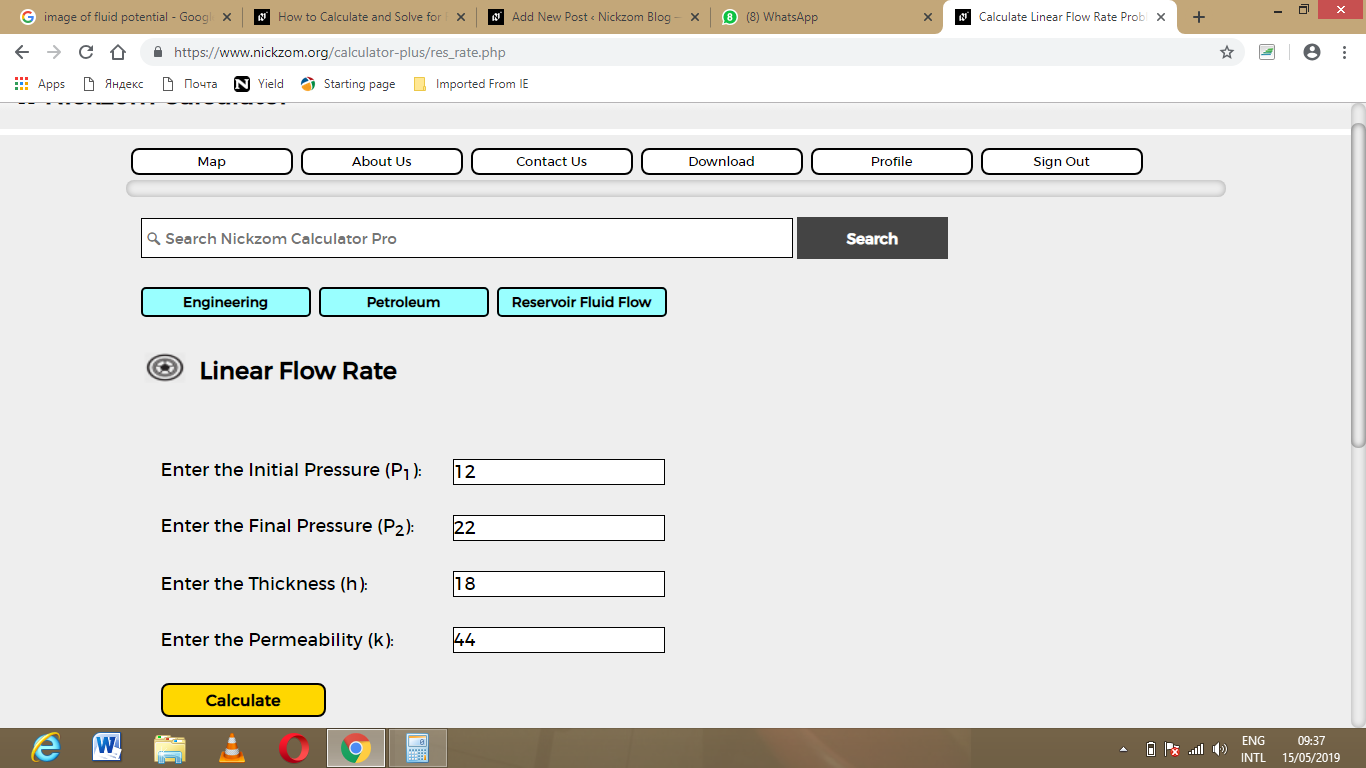Finally, Click on Calculate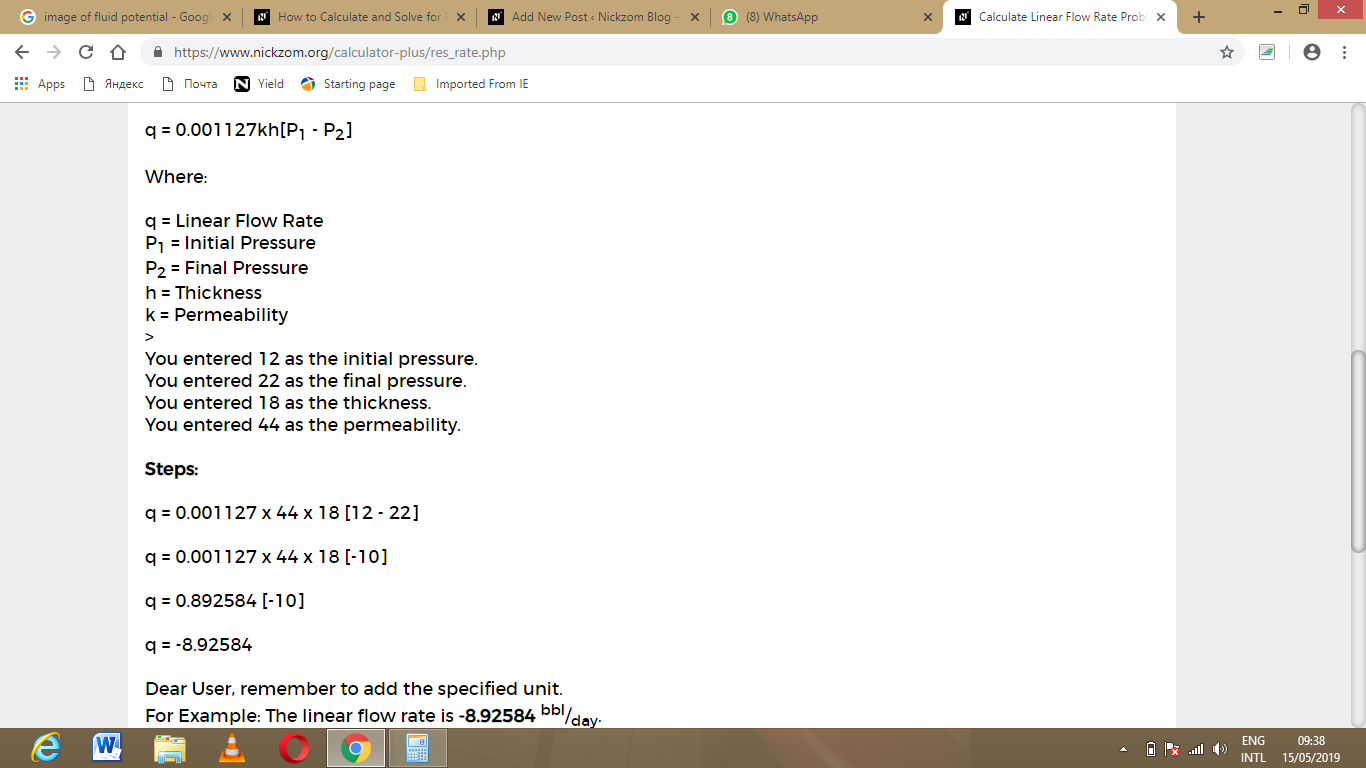As you can see from the screenshot above, Nickzom Calculator – The Calculator Encyclopedia solves for the linear flow rate and presents the formula, workings and steps too.